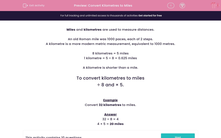# Convert Kilometres to Miles

In this worksheet, students must convert distances from kilometres to miles.Key stage:  KS 2

Curriculum topic:   Maths and Numerical Reasoning

Curriculum subtopic:   Metric & Imperial

Difficulty level:#### Worksheet Overview

Miles and kilometres are used to measure distances.

An old Roman mile was 1000 paces, each of 2 steps.

A kilometre is a more modern metric measurement, equivalent to 1000 metres.

8 kilometres = 5 miles

1 kilometre = 5 ÷ 8 = 0.625 miles

A kilometre is shorter than a mile.

To convert kilometres to miles

÷ 8 and × 5.

Example

Convert 32 kilometres to miles.